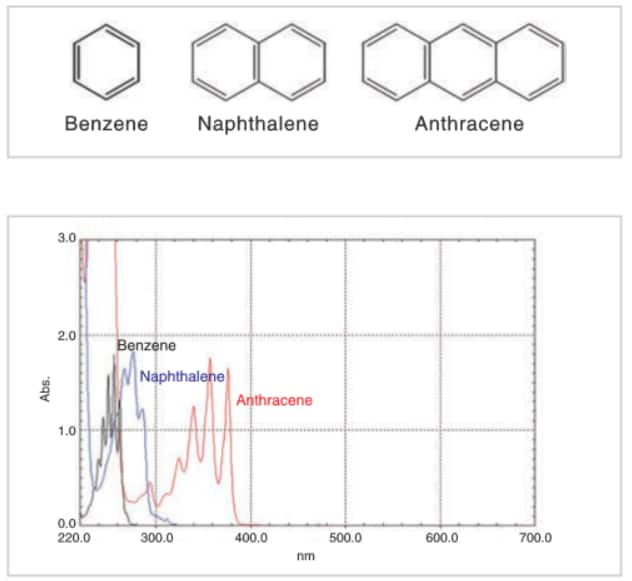# What is the relationship between absorption spectroscopy and polyaromatic ring structure?There are many organic compounds that have conjugated double bond systems (hereafter referred to as “conjugated systems”), in which every other bond is a double bond. These conjugated systems have a large influence on peak wavelengths and absorption intensities.

The top figure shows the structures of benzene, naphthalene, and anthracene. The bottom figure shows the absorption spectra obtained by dissolving these compounds in ethanol and analyzing the resulting solutions. The concentrations were adjusted so that the absorption intensities of the components were roughly the same. It can be seen in the figure that peak wavelengths tend to be shifted toward the long wavelength region as the conjugated system gets larger.

Why, then, does the peak wavelength tend to be shifted toward the long wavelength region as the size of the conjugated system increases? Let us consider the relationship between the energy of light and the movement of electrons.

Light exhibits properties of both waves and particles (photons). The energy of one photon is expressed as hc/λ, where h is Planck’s constant, c is the speed of light, and λ is the wavelength. Absorption in the ultraviolet and visible regions is related to the transition of electrons. “Transition” refers to the switching of an electron from one state of motion to another. The state of motion of the π electrons in the conjugated system changes more easily than that of the σ electrons that form the molecular frameworks. If a photon collides with a π electron, that π electron readily changes to a different state of motion. This is true even if the photon has only a small amount of energy. The π electrons in relatively large conjugated systems are more easily affected by low-energy photons. Transition expresses the way that the energy of photons is absorbed by electrons. If a photon has a relatively small amount of energy, the value of hc/λ for that photon is relatively small, and therefore the value of λ is relatively large. λ is observed as the absorption wavelength and so, if there is a conjugated system, peaks tend to appear in regions where λ is large, i.e., the long wavelength region.

Back to Index